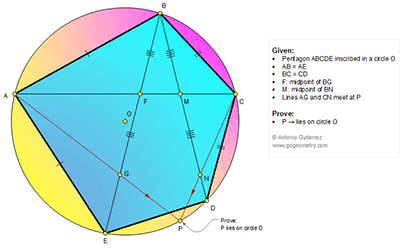## Friday, July 24, 2015

### Geometry Problem 1141: Inscribed Pentagon, Circle, Triangle, Congruence, Midpoint, Concurrency

Geometry Problem. Post your solution in the comments box below.
Level: Mathematics Education, High School, Honors Geometry, College.

Click the diagram below to enlarge it.#### 1 comment:

1.Consider Tr.ABC , lets assume Angle ACB = x and Angle BAC =y ,
We get Angle ABC = 180 - (x+y)

Tr. AFB and Tr. BMC are similar
AF/FB = BM/CM
Angle AFB = Angle BMC = 180 -x - y

Now consider Tr.AFG and Tr. NMC
AF/FG = MN/CM, angle AFG = angle NMC = x + y ,
Angle AGF = Angle MCN , by AA similarity Tr.AFG is similar to Tr. APC
Angle APC = Angle AFG = x + y
Quad. ABCP is cyclic hence P lies on circle O.
QED